> 文档中心 > ＜算法与数据结构＞详解贪心策略之最小生成树的Prime算法的设计与实现

# ＜算法与数据结构＞详解贪心策略之最小生成树的Prime算法的设计与实现

🎉情人节快乐！

🎉 没有对象就搁这儿好好学习，有对象了就为了对象好好学习

🎉写在前面

🎉目录

Prime算法思想

# 浅析最小生成树

G=(V,E)是无向连通带权图。E中每条边(v,w)的权为c[v][w]

# Prime算法思想

G=(VE)是无向连通带权图，V={1,2,…,n}

# 此算法核心部分

## 实现思路

tips:onTreet 属性是布尔类型，为true时该结点在“树”上

# 构造实例

Prim算法对如图所示的无向连通带权图构造一棵最小生成树。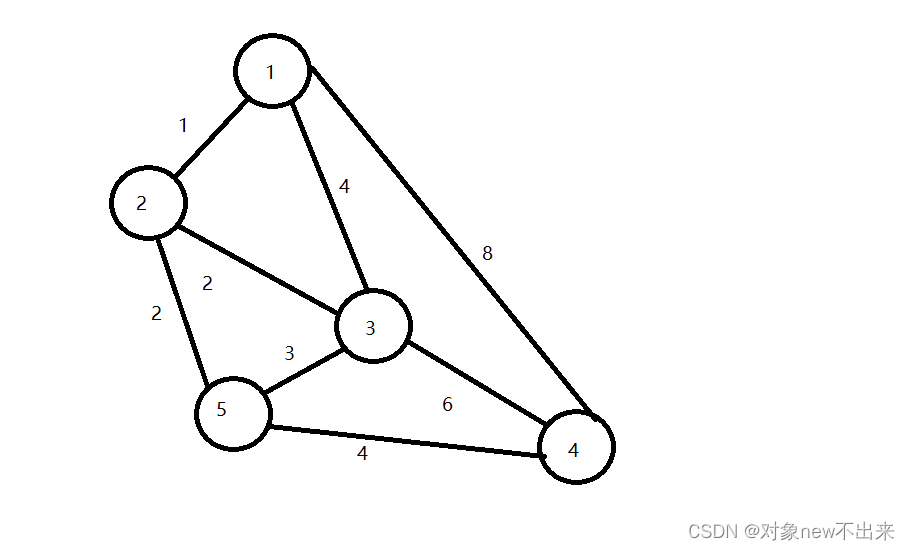# 构造过程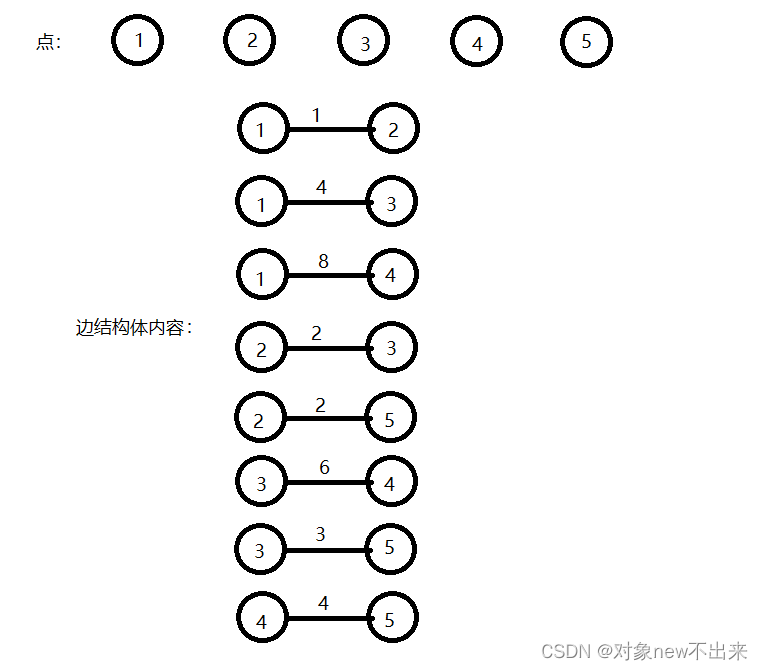点和边结构体数组图示如上所示，我们需要的最终效果为下图所示：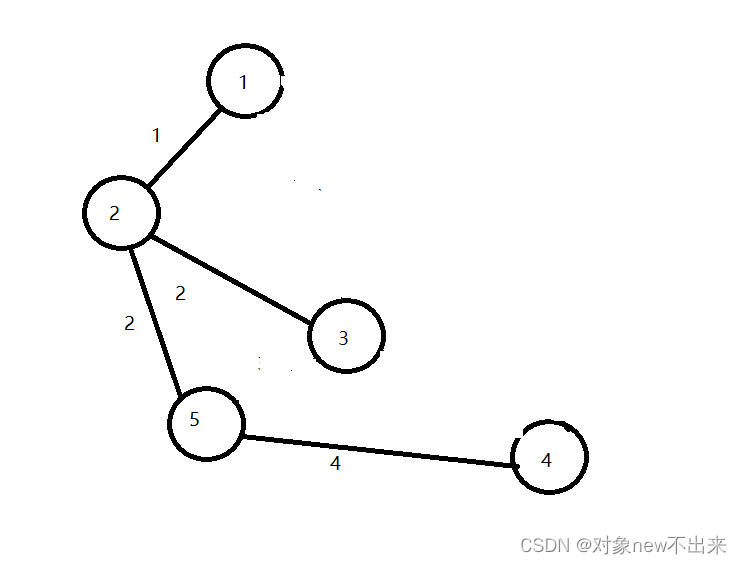# 代码详解

``#include using namespace std;struct Node {int ID;//结点序号bool OnTree;//是否属于最小生成树};struct LS {int N1, N2; int V; bool OnTree;//OnTree用于判断此边是否在“树”上LS(int n1, int n2, int v) {N1 = n1; N2 = n2; V = v; OnTree = false;//N1,N2为边左右连接点，v是边的权值}};Node A[] = { {1,false}, {2,false}, {3,false}, {4,false}, {5,false} };//点结构体数组LS L = { LS(1,2,1),LS(1,3,4) ,LS(2,3,2),LS(2,5,2),LS(4,5,4),LS(3,4,6),LS(3,5,3),LS(1,4,8)};//边结构体数组bool FindOne(LS L ,Node A[]) {//布尔类型int m = 0;for (int i = 0; i < 5; i++)if (L.N1 == A[i].ID && A[i].OnTree) m++;for (int i = 0; i < 5; i++)if (L.N2 == A[i].ID && A[i].OnTree) m++;return m ==1;//只有N1和N2的一个连接到了在“树”上的结点才为真}int main(){A.OnTree = true;for (int i = 0; i < 5; i++) {int p = 0;for (int j = 0; j < 8; j++) {if (FindOne(L[j], A)) {p = j; break;}}for (int i = 0; i < 8; i++) {if (FindOne(L[i], A))if (L[i].V < L[p].V) p = i;}L[p].OnTree = true;//选中的边设置为在“树”上 //将边的连接点放在“树”上for (int i = 0; i < 5; i++) {if (L[p].N1 == A[i].ID) A[i].OnTree = true;if (L[p].N2 == A[i].ID) A[i].OnTree = true;}}    //输出最小生成树所有边for (int i = 0; i < 8; i++) {cout << L[i].OnTree;}}``

``bool FindOne(LS L ,Node A[]) {//布尔类型int m = 0;for (int i = 0; i < 5; i++)if (L.N1 == A[i].ID && A[i].OnTree) m++;for (int i = 0; i < 5; i++)if (L.N2 == A[i].ID && A[i].OnTree) m++;return m ==1;//只有N1和N2的一个连接到了在“树”上的结点才为真}调用方法 ： FindOne(L[j], A)``

# 调试结果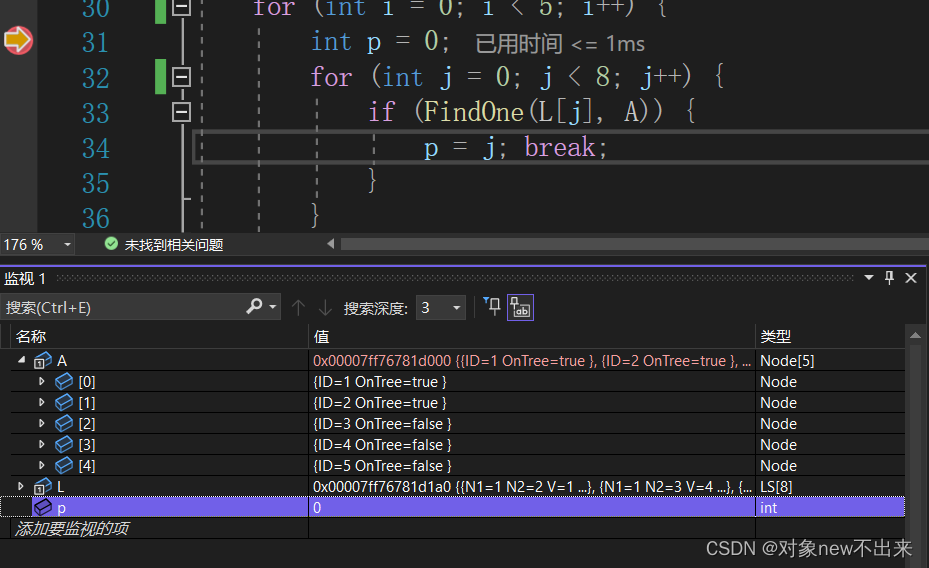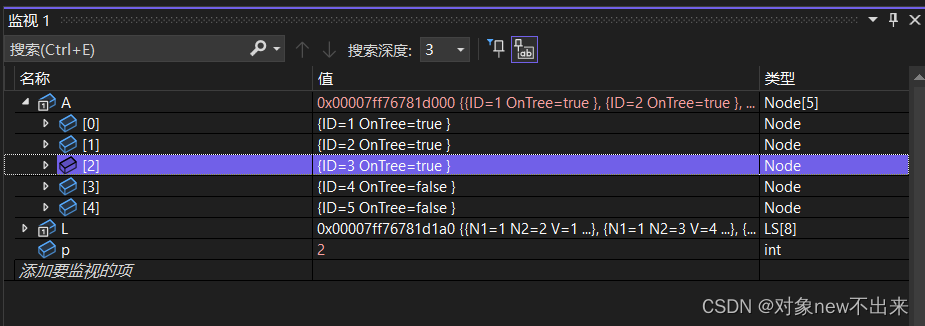​​​​​​第三次循环，p为3，同理，ID5会变成true；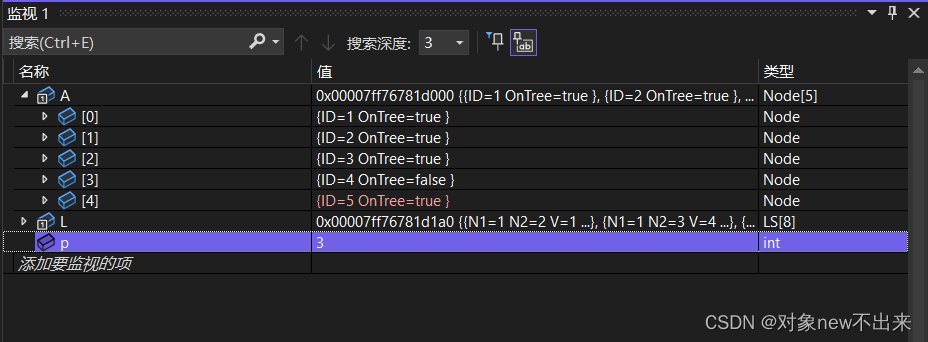接下来重复上面的过程，直到没有满足条件的边，循环结束；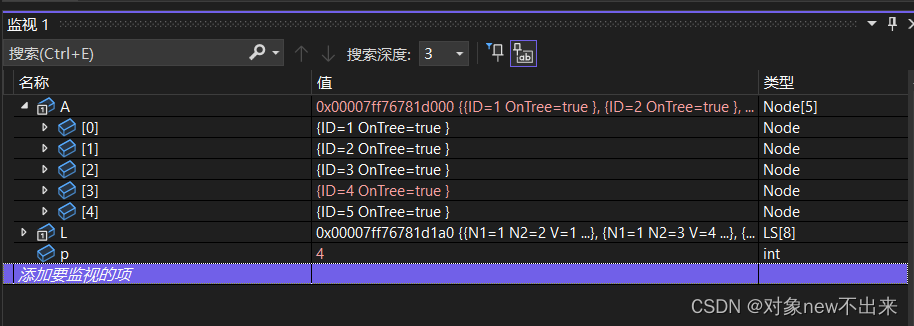最后就是输出所有在“树”上的边了，数组中为1的边就是被选中的边，这样清晰的得到了最终的最小生成树了。# 总结

Prime算法属于贪心算法的一种，尽情的找到权值最小的边并连接到一起，最小生成树的算法分享与实现圆满完成了，希望对大家有实质性的帮助，期待你们的支持，下篇文章再见吧！